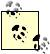# Exploring VBAs Built-in Functions

### Exploring VBA s Built in Functions

Problem

You need to get familiar with VBA's built-in functions and use them in your code, but you're not sure where to begin.

Solution

Check out the Visual Basic Language Reference in the VBA help guide. There's a section in the language reference entitled "Functions," which includes an alphabetically organized list of built-in functions. For your convenience, I've summarized some of the more commonly used functions for scientific and engineering calculations in Table 2-2.

Table 2-2. Some useful VBA fuctions

Function

Comment

Abs(number)

Returns the absolute value of the number argument. The data type returned is the same as that of the argument.

Atn(number)

Returns the arctangent of the number argument. The returned data type is a Double. The result is in radians from -p/2 to p/2.

Cos(number)

Returns the cosine of the number argument, which represents an angle in radians. The return type is a Double in the range from -1 to 1.

Exp(number)

Returns e raised to the number power. The return type is a Double.

Int(number)

Returns the integer part of number, where number is a decimal number. The return type is an Integer. Int essentially truncates the number. To round a number use the Round function.

Log(number)

Returns the natural logarithm (to base e) of number. The return type is a Double. To calculate the base 10 log of a number, use the expression Log(number) / Log(10).

Round(number)

Returns number rounded to the nearest integer. The return type is an Integer.

Round(number, n)

Returns number rounded to the nearest decimal place specified by n. The return type is a Double.

Sgn(number)

Returns the sign of number. Sgn returns -1 if number is negative, 1 if number is positive, and 0 if number equals zero.

Sin(number)

Returns the sine of number, which represents an angle in radians. The return type is a Double in the range from -1 to 1.

Sqr(number)

Returns the square root of number. The return type is a Double. Taking the Sqr of a negative number causes an error.

Tan(number)

Returns the tangent of number, which represents an angle in radians. The return type is a Double.

Discussion

You can call VBA functions in your own expressions, as illustrated in Example 2-16.

Example 2-16. Calling VBA functions

 ```num1 = 1 - cos(1.571) num2 = 1 - Atn(a/b) ```

You need not hardcode arguments as shown in the first statement. You can use an expression like that shown in the second statement as an argument to a function so long as the expression evaluates to an acceptable value permitted by the particular function.

As discussed in Recipe 1.10, Excel has a great many useful functions that you can use in conjunction with the VBA functions discussed here. You can actually call Excel's built-in functions from within a VBA procedure. See Recipe 2.13 for more information on calling Excel functions from VBA code. You have to be a little careful when working with both VBA and Excel functions. VBA and Excel both have some functions that perform the same (or a similar) operation, but in some cases functions with the same name are different, and this can be confusing. For example, both Excel and VBA have a Log function; however, the VBA version of the function returns the base e logarithm while the Excel version returns the base 10 logarithm unless you specify otherwise. This is just something to keep in mind when mixing functions.Some programming languages have a function that raises a number to some exponent. VBA does not; however, you can use the ^ operator to raise a number to an exponent just as you do when raising a number to a power in an Excel formula. For example, the expression num^3 raises the variable num to the third power. You can also include an expression as the exponent, as in num^(k*1.3). See Recipes 1.8 and 1.9 for more information.Excel Scientific and Engineering Cookbook (Cookbooks (OReilly))
ISBN: 0596008791
EAN: 2147483647
Year: N/A
Pages: 206
Authors: David M Bourg